﻿ MULT - Multiplier File
Online Guide to MODFLOW

MULT - Multiplier File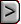MULT - Multiplier File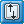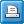MULT - Multiplier FileAbbreviation in Name file

MULT

Purpose

The Multiplier File  is used to specify multiplier arrays which can be used to calculate layer variables from parameter values.

Documentation

 • Harbaugh, A.W., Banta, E.R., Hill, M.C., and McDonald, M.G., 2000, MODFLOW-2000, the U.S. Geological Survey modular ground-water model -- User guide to modularization concepts and the Ground-Water Flow Process: U.S. Geological Survey Open-File Report 00-92, 121 p.
 • ofr-00-92-errata.pdf
 • Harbaugh, A.W., 2005, MODFLOW-2005, the U.S. Geological Survey modular ground-water model -- the Ground-Water Flow Process: U.S. Geological Survey Techniques and Methods 6-A16. https://pubs.usgs.gov/tm/2005/tm6A16/
 • Hanson, R.T., Boyce, S.E., Schmid, Wolfgang, Hughes, J.D., Mehl, S.M., Leake, S.A., Maddock, Thomas, III, and Niswonger, R.G., 2014, One-Water Hydrologic Flow Model (MODFLOW-OWHM): U.S. Geological Survey Techniques and Methods 6–A51, 120 p., https://dx.doi.org/10.3133/tm6A51.

Supported in

 • MODFLOW-2000
 • MODFLOW-2005
 • MODFLOW-LGR
 • MODFLOW-CFP
 • MODFLOW-NWT
 • MODFLOW-OWHM

Other Notes

Multiplier arrays are used in the ADV2, ETS, EVT, HUF2, KDEP, LPF, LVDA, RCH, and UPW input files.

Input Instructions

Input to define multiplier arrays is read from the file that is specified with "MULT" as the file type. Multiplier arrays can be used to calculate layer variables from parameter values.

FOR EACH SIMULATION

Data Set 0

[#Text]

Item 0 is optional—“#” must be in column 1. Item 0 can be repeated multiple times.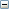Text
 Text—is a character variable (199 characters) that starts in column 2. Any characters can be included in Text. The “#” character must be in column 1. Except for the name file, lines beginning with # are restricted to these first lines of the file. Text is printed when the file is read.

Data Set 1

NML [MULTPRINT]Explanation of variablesNML
 NML—is the number of multiplier arrays to be defined.  In MODFLOW-2000, the maximum number of multiplier arrays is 1000.MULTPRINT
 an optional print flag that when set >0 writes all multiplier arrays, vectors, or scalars to file called “MULT_Arrays.txt.” This will not occur if MULTPRINT is not present or set to <1. MULTPRINT is only available in MODFLOW-OWHM.

Repeat Item 2 with Items 3 or 4 or 5 until NML multiplier arrays, vectors, or scalars have been defined.

Data Set 2

MLTNAM [ FUNCTION] [EXPRESSION]MLTNAM
 MLTNAM—is the name of a multiplier array. This name can consist of 1 to 10 characters and is not case sensitive. That is, any combination of the same characters with different case are equivalent. The name “NONE” should not be used for a multiplier array because it is a reserved word. For further restrictions, see Algebraic_Expression.FUNCTION
 The optional keyword, FUNCTION, indicates that the multiplier array will be constructed from other multiplier arrays that have already been defined. Construction is by arithmetic combinations of the multipliers; see the explanation below for variable op1, op2, op3 ....EXPRESSION
 is an optional keyword, which indicates that the multiplier array will be constructed from other multiplier arrays, vectors, or scalars that have already been defined. Construction is different from the FUNCTION keyword in that it follows mathematical order of operations with parenthetic support. See explanation for variable Algebraic_Expression.

Data Set 3

[RMLT(NCOL,NROW)] - U2DREL

If Item 2 does not contain the optional FUNCTION key word or optional EXPRESSION key word, read item 3. Item 2 cannot have both key words FUNCTION and EXPRESSION at the same time.RMLT
 RMLT—is a multiplier array.

Data Set 4

[MLTNAM1 [op1 MLTNAM2] [op2 MLTNAM3] [op3 MLTNAM4] ... ] [IPRN]

Item 4 is only read if Item 3 contains the optional FUNCTION key word.Explanation of variablesMLTNAM1, op1, MLTNAM2, op2, MLTNAM3, ...
 MLTNAM1, MLTNAM2, MLTNAM3, ...—are the names of multiplier arrays that have already been defined. op1, op2, op3, ...—are arithmetic operators used to define a multiplier array based on other multiplier arrays. Each operator can be either “+”, “-”, “*”, or “/”. Operations are applied from left to right to each array element. The operators must be separated from the multiplier array names by at least one space. In MODFLOW-OWHM, "^" can be used as the exponentiation operator.IPRN

IPRN is a flag that indicates if the array defined in Item 4 should be printed (written to the listing file) after it has been generated and a code for indicating the format that should be used when it is printed.

 • If IPRN < 0, printing of the multiplier array is suppressed.
 • If IPRN ≥ 0, The array is printed using the formats defined for IPRN in the U2DREL Array Reading Utility Module.

Data Set 5 (Only supported in MODFLOW-OWHM)

[Algebraic_Expression]

Item 5 is only read if Item 3 contains the optional EXPRESSION key word. It is a single mathematical expression composed of previously defined multiplier arrays.Algebraic_Expression
 Algebraic_Expression is a single line with a maximum length of 500 characters that contains an algebraic expression composed of multiplier arrays, vectors, or scalars that have already been defined (that is, MLTNAM). The expression is evaluated by following mathematical order of operations (also called operator precedence). The expression calculates the result using the following algebraic operators “+,” “–,” “*,” “/,” “^,” and parentheses “(“, “)” for multinomial expressions. Decimal (for example, 1.4 and 2.33) and integer (for example, 1 and 2) numbers can be included in the expression, but NOT exponential notation (for example, 1E–5). The expression parser has an additional set of keywords, that CAN NOT appears anywhere in a variable names that is used in the expression (that is, MLTNAM). The keywords operate on what is inside the ( ) and are as follows: ABS() for absolute value ( |x| ) EXP() for solving the natural exponential function ( ex ) LOG() for solving the natural logarithm ( loge ) L10() for solving the base 10 logarithm ( log10 ) NEG() for multiplying the function by negative one ( NEG(x) = –x ) SQRT() for solving the square root ( √ ) of positive values only

Example Multiplier Array Input Using the FUNCTION and EXPRESSION Keywords in MODFLOW-OWHM.

The following two example inputs illustrate the use of the FUNCTION and EXPRESSION keywords to construct a multiplier array from other multiplier arrays. In the first example, a model layer that has 5 rows and 4 columns has six multiplier arrays, vectors, or scalars to be defined, and, accordingly, the first line of the file contains “6.” The first two arrays (named M1 and M2) are read using the U2DREL utility array reader (Item 3), the third array (named POW) is a multiplier array set to the number 2. The fourth array (named M4) is defined by using the FUNCTION key word, which follows left to right order of operations. This results in calculating M4 as the sum of M1 and M2, which is then raised to power POW (that is, (M1+M2)POW). Note that the "^" operator is only  supported in MODFLOW-OWHM. The fifth matrix (named M5) is defined by using the EXPRESSION key word, which follows mathematical order of operations and produces an identical multiplier to M4. The sixth matrix (named M6) is defined by using the EXPRESSION key word that calculations M2POW first and then sums the result with M1.

The second example is a more complex application of the EXPRESSION key word that constructs a multiplier matrix composed of a weighted power mean,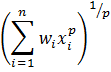and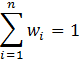, of two multiplier matrices (n = 2). In this second example there are eight multiplier arrays, vectors, or scalars to be defined, and accordingly the first line of the file contains “8”. The first two multiplier arrays, vectors, or scalars (named X1 and X2) define x1 and x2. The third multiplier array (named POW) defines the power mean power, p, which for this case is –1 to represent a harmonic mean. The fourth multiplier matrix is a matrix composed of all ones. The fifth multiplier (named W1) is the first weight, w1, read from a free formatted external file. The sixth multiplier (named W2) is the second weight, w2 = 1 – w1, which is constructed using the FUNCTION key word (left to right order of operations). The seventh multiplier (named PowerMean1) solves the power mean using W2 with the EXPRESSION key word. The eighth multiplier (named PowerMean2) solves the power mean without using W2 via the EXPRESSION key word.

EXAMPLE 1

7

M1

INTERNAL 1.0 (4F6.0) 0

1.0 1.1 1.2 1.3

1.0 1.1 1.2 1.3

2.0 2.2 2.4 2.6

2.0 2.2 2.4 2.6

1.0 1.1 1.2 1.3

M2

INTERNAL 1.0 (4F6.0) 0

5.0 5.1 5.2 5.3

5.0 5.1 5.2 5.3

6.0 6.1 6.2 6.3

6.0 6.1 6.2 6.3

5.0 5.1 5.2 5.3

POW

CONSTANT 2.0

M3 FUNCTION

M1 + M2

M4 FUNCTION

M1 + M2 ^ POW

M5 EXPRESSION

(M1+M2)^POW

M6 EXPRESSION

M1+M2^POW

The resulting values for multiplier M3 are:

6.0 6.2 6.4 6.6

6.0 6.2 6.4 6.6

8.0 8.3 8.6 8.9

8.0 8.3 8.6 8.9

6.0 6.2 6.4 6.6

The resulting values for multipliers M4 and M5 are:

36.00 38.44 40.96 43.56

36.00 38.44 40.96 43.56

64.00 68.89 73.96 79.21

64.00 68.89 73.96 79.21

36.00 38.44 40.96 43.56

The resulting values for multiplier M6 is:

26.00 27.11 28.24 29.39

26.00 27.11 28.24 29.39

38.00 39.41 40.84 42.29

38.00 39.41 40.84 42.29

26.00 27.11 28.24 29.39

EXAMPLE 2

9

X1                                                   #FIRST NUMBER X1

CONSTANT 25.

X2                                                   #SECOND NUMBER X2

CONSTANT 0.01

POW                                                  #POWER USED IN POWER MEAN, p

CONSTANT -1.0

ONES                                                 #MATRIX OF ALL ONES

CONSTANT 1.0

W1

OPEN/CLOSE FIRST_WEIGHT.TXT 1.0 (FREE) -1

W2 FUNCTION                                          #CALCULATE SECOND WEIGHT AS

ONES - W1

PowerMean1 EXPRESSION                                #POWER MEAN

(W1*X1^POW + W2*X2^POW)^(ONES/POW)

PowerMean2 EXPRESSION                                #POWER MEAN (DOES NOT REQUIRE W2)

(W1*X1^POW + (ONES-W1)*X2^POW)^(ONES/POW)

KeywordEx EXPRESSION                                 #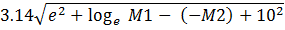3.14 * SQRT( EXP(POW) + LOG(M1) - NEG(M2) + 10^POW )